# NCERT Solutions for Class 10 Maths Chapter 14 - Statistics

Share

NCERT Mathematics Solutions for Class 10th Chapter 14- Statistics are provided here in order to help students in getting through with the basic concepts of the topics and check the step-wise solutions for the questions that are asked at the end of each chapter of NCERT textbook. For more effective preparation, you will find these Solutions covering the complete syllabus, error-free and based on the current CBSE curriculum. Avail these NCERT Solutions at free of cost.

Chapter 14 Statistics holds a total of weightage of 11 marks in the final examination and deals with concepts related to study of three measures which are mean, median and mode from ungrouped data to that of grouped data. Learn the concept of Cumulative Frequency, Cumulative Frequency distribution, and how to draw Cumulative Frequency Curves and what you understand by the term Ogives

## NCERT Solutions for Class 10 Maths Chapter 14 - Statistics

Exercise 14.1
• Exercise 14.1
• Exercise 14.2
• Exercise 14.3
• Exercise 14.4
Class 10th|NCERT - MathematicsChapter 14 - Statistics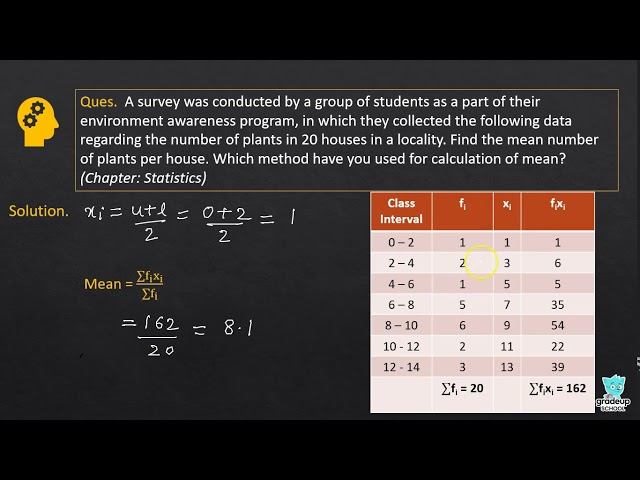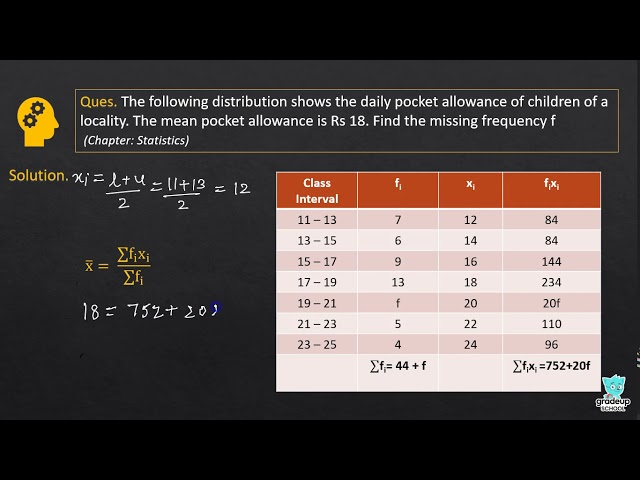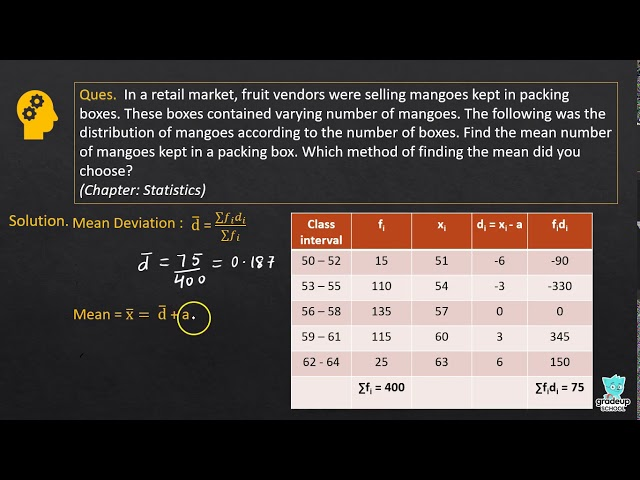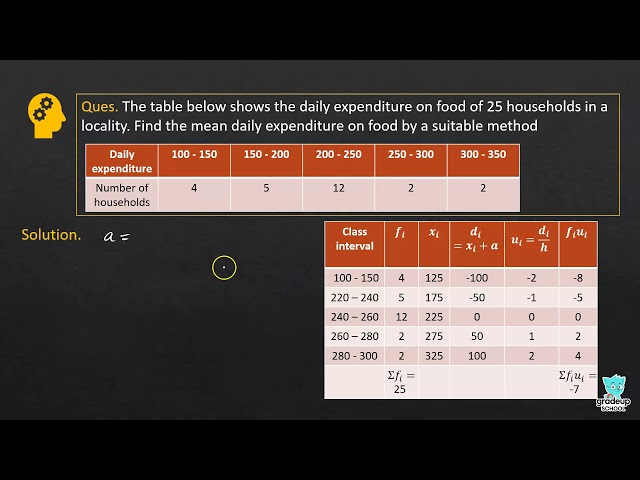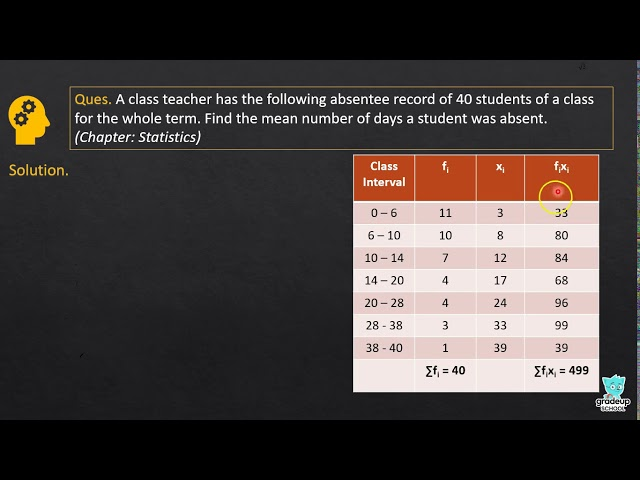NCERT - MathematicsClass 10th , NCERT
 Chapter 1 - Real Numbers Chapter 2 - Polynomials Chapter 3 - Pair of Linear Equations in Two Variables Chapter 4 - Quadratic Equations Chapter 5 - Arithmetic Progressions Chapter 6 - Triangles Chapter 7 - Coordinate Geometry Chapter 8 - Introduction to Trigonometry Chapter 9 - Some Applications of Trigonometry Chapter 10 - Circles Chapter 11 - Constructions Chapter 12 - Areas Related to Circles Chapter 13 - Surface Areas and Volumes Chapter 14 - Statistics Chapter 15 - Probability